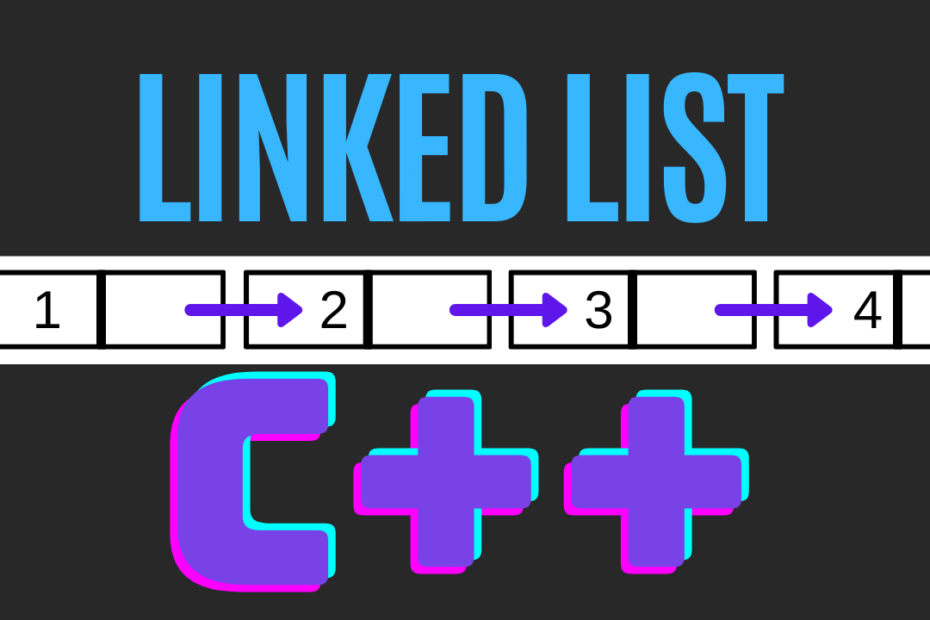# Linked List Code in C++

• DSA

Linked List is one of the most important data structures and understanding how it works is easier than you think, this post explains the Linked List code in C++.

## Important Operations on a Linked List

As you can see below we use a separate class for Linked List this helps us to clearly define and manage all the functions and data members easily, also have a look at the constructors, the initialization is done automatically with the help of them.

The main functions/operations of a Linked List are:

• Insert() – insert a node at a particular position in the list
• Delete() – remove/delete a node at an index
• Length() – returns the length of the Linked List
• Display() – display’s the contents of the Linked List

Finally, we use the ~Destructor to delete everything after we’re done

### What is Destructor?

A Destructor is the opposite of the constructor like we use a constructor to initialize objects in a similar way we use a destructor to delete the Objects of a class.

## Code

``````#include <iostream>
using namespace std;

class Node
{
public:
int data;
Node *next;
};

{
private:
Node *first;

public:
LinkedList() { first = NULL; }
//destructor
void Display();
void Insert(int index, int x);
int Delete(int index);
int Length();
};

{
Node *last, *t;
int i = 0;

first = new Node;
first->data = A;
first->next = NULL;
last = first;

for (i = 1; i < n; i++)
{
t = new Node;
t->data = A[i];
t->next = NULL;
last->next = t;
last = t;
}
}

{
Node *p = first;
while (first)
{
first = first->next;
delete p;
p = first;
}
}

{
Node *p = first;

while (p)
{
cout << p->data <<" " ;
p = p->next;
}
cout << endl;
}

{
Node *p = first;
int len = 0;

while (p)
{
len++;
p = p->next;
}
return len;
}

{
Node *t, *p = first;

if (index < 0 || index > Length())
return;
t = new Node;
t->data = x;
t->next = NULL;

if (index == 0)
{
t->next = first;
first = t;
}
else
{
for (int i = 0; i < index - 1; i++)
p = p->next;
t->next = p->next;
p->next = t;
}
}

{
Node *p, *q = NULL;
int x = -1;

if (index < 1 || index > Length())
return -1;

if (index == 1)
{
p = first;
first = first->next;
x = p->data;
delete p;
}
else
{
p = first;
for (int i = 0; i < index - 1; i++)
{
q = p;
p = p->next;
}
q->next = p->next;
x = p->data;
delete p;
}
return x;
}

int main()
{
int A[] = {1, 2, 3, 4, 5};
l.Insert(3, 10);
l.Display();
l.Delete(4);
l.Display();
cout<<l.Length()<<endl;
cout<<l.Length()<<endl;
return 0;
}``````

## Output

First, we pushed 1,2,3,4,5 then inserted 10, later deleted 10 and then we checked the length and at last, we use the destructor to delete the Linked List i.e the final length becomes zero as the Linked List is deleted

``````1 2 3 10 4 5
1 2 3 4 5
5
0``````

This post is part of my #30DaysChallenge to write a blog post every day on what I learn. Happy Coding ~ Abhiram Reddy